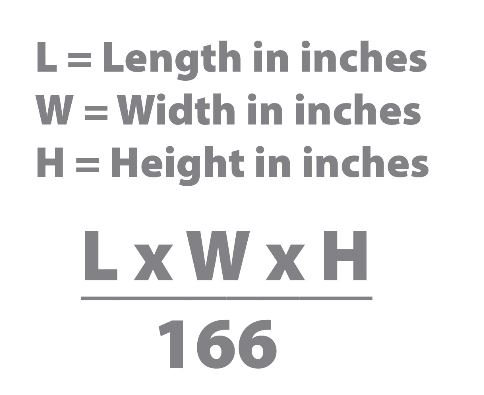# How to Calculate Dimensional Weight

## What is dimensional weight?

Dimensional weight reflects package density, which is the amount of space a package occupies in relation to its actual weight.

Billable weight is the weight used to calculate your rate. For domestic and international services, the billable weight will either be the dimensional weight or the actual weight of your package (whichever number is greater).### How is dim weight calculated?

Dimensional weight is calculated based on the length, height, and width of the package. The dimensional weight becomes the billable weight when the dimensional weight of your package exceeds its actual weight.

Follow these easy steps to calculate dimensional weight:

1. Calculate the cubic size of your package in inches. Multiply the length by width by height of your package. Round each measurement to the nearest whole inch. The resulting total is the cubic size of your package.
2. Determine actual weight. Use any standard scale to determine the actual weight of your package. Increase any fraction to the next whole pound.
3. Determine dimensional weight. Divide the cubic size of your package in inches by 166 (for shipments within the U.S. and shipments between the U.S. and Puerto Rico) or 139 ( for international shipments).
4. Determine billable weight. Compare the package’s actual weight to its dimensional weight. The larger of the two weights is the billable weight and should be used to calculate the rate.
5. For multiple-package shipments, total the billable weight of all packages in the shipment.

This method is used to calculate Air and Ocean freight charges billed by shipping companies. When a package is delivered to a shipping company, it is weighed and the volume is determined. The charge of the shipment is based on whatever is higher.

## Sample Calculation of chargeable weight

Ship Method / Destination: Ground / Domestic
Actual Weight: 21 pounds
Length: 30 inches
Width: 12 inches
Height: 12 inches
Cubic Size Calculation: 30 x 12 x 12 = 4,320 cubic inches
Dimensional Weight Calculation: 4,320/166 = 26 pounds

In this first example, because it is a domestic shipment, we multiply the total cubic size in inches by 166. In doing so, we find out that the dimensional weight is 26 pounds, which is greater than the actual weight.

However, because the package is being shipped via ground service and is less than 5,184 cubic inches, the dimensional weight is not applied. So in this case, the actual weight of 21 lbs. becomes the billable weight.

Ship Method / Destination: Air / International
Actual Weight: 18 pounds
Length: 24 inches
Width: 12 inches
Height: 12 inches
Cubic Size Calculation: 24 x 12 x 12 = 3,456 cubic inches
Dimensional Weight Calculation: 3,456/139 = 25 pounds